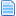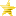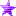# Math for the Algebraically Challenged (Read 11926 times)

Started by Bronko, November 20, 2005, 04:19:10 pm

#### FrozenDelight##### Re: Math for the Algebraically Challenged
#21  November 22, 2009, 05:54:50 pm
••• Why can't I upload a Profile Pic?!

#### Bronko##### Re: Math for the Algebraically Challenged
#22  November 25, 2009, 03:29:47 pm
••• Just a butcher on a mission.
For now I'm going to see if I can find the images again and re-upload.

#### Seravy##### Re: Math for the Algebraically Challenged
#23  October 21, 2011, 01:17:24 am
••There is a mistake around here :
Quote
Time^2 is just another notation for Time*Time. The bigger the exponent, the faster the graph curves upward.  And just as the inverse of multiplication is division, the inverse of an exponent is a logarithm.
Logarithm base 2 would be the inverse of 2^Time, not Time^2.
Time^2 is the square of Time, and the inverse of that is Square Root, so the graph below is Sqrt(time) and not log(2,time).

For comparison,
log(2,64)=6 (because 2^6=2*2*2*2*2*2=64)
log(2,1024)=10  (because 2^10=2*2*2*2*2*2*2*2*2*2=1024)
log(2,65536)=16 (because 2^16=65536)
sqrt(64)=8 (because 8^2=8*8=64)
sqrt(1024)=32. (because 32^2=32*32=1024)
sqrt(65536)=256. (because 256^2=256*256=65536)

As you can see, logarithm grows a lot slower than square root does.
Other than that, nice guide, math is indeed often useful when coding things in Mugen, and there are way too many people not using it.

#### Bronko##### Re: Math for the Algebraically Challenged
#24  March 08, 2012, 09:27:32 pm
••• Just a butcher on a mission.
I've neglected this thread for far too long and it could really use a bit of a rewrite.  Thanks for catching that Seravy I don't know what I was thinking when I wrote that section.
Anyways I've written up a fairly detailed explanation of common trigonometric functions, hopefully there's one person who won't tl;dr it.

If you want to understand how sine, cosine, and tangent work you need to understand a few fundamental things about circles and triangles.

Consider a whole circle.  The circumference is PI * 2 * radius.  For the sake of this explanation we will use what is a called a unit circle, which is a circle with radius 1
Which means our circumference is simply PI * 2.  We also have a unit of angle measurements called radians, which are simply relating angles to the length of an arc on our unit circle.  That is to say 360 degrees (a full circle) is equal to 2*PI radians.
In mugen, sin, cos, and tan use radians as parameters, not degrees.  Therefore, before you do any calculation you must remember to use the correct conversion.

What are sin, cos, and tan?
These are ratios of the sides of a right triangle.  As long as we know the angles of a triangle, and one angle on that triangle is 90 degrees (PI/2 radians) we can know for certain the ratios of the lengths of all three sides of the triangle.
For example, if we have a triangle with two angles of 45 degrees and one angle of 90 degrees (your Geometry teacher will call this a 45/45/90 triangle) then the two sides near the right angle will be X and the hypotenuse will be of length X * square root of 2.  It doesn't matter how big the triangle is, those ratios will be constant as long as the angles don't change.
So how are these ratios defined?

Most people in school will learn SOHCAHTOA
Say it repeatedly until you remember it.

SOH = Sine,  Opposite over Hypotenuse
CAH = Cosine, Adjacent over Hypotenuse
TOA = Tangent, Opposite over Adjacent

Naturally in a right triangle, the hypotenuse refers to the side directly opposite of the right angle.  So in our 45/45/90 example, we can take the sine of one of the 45 degree angles, and that'll give us 1/sqrt(2)
Plug in sin(45) in a calculator and you'll get that answer.

If you know the lengths of a right triangle, but you don't know the other two angles, you can use inverse trigonometry and input your ratio in order to get the angle, asin, acos, and atan.

I still don't get it.
If you want to imagine sin and cos graphically, imagine the unit circle in your head.  You know, the circle with radius 1.  Now imagine that circle is on a graph, with the center at 0,0.  That means that 0 degrees on the circle (furthest right) is at point 1,0
If you want to know the position of any point in the circle, the X coordinate is cos and the Y coordinate is sin.  So sin(0) is 0 and cos(0) is 1.  At the top of the circle, 90 degrees, the circle is at 0,1.  So sin(90) = 1 and cos(90) = 0.  Looking at it this way, you can observe a few interesting things about sin and cos

- Because the unit circle only has a radius of 1, sin and cos will only go from -1 to 1
- sin and cos repeat themselves after going a full 360 degrees (2*PI) this is also called the period of the function.
- cosine is just a sine that's been shifted by 90 degrees (PI / 2)

`[State 3150, Fulcrum] ; Spawn the center of our "circle"type = Helpertrigger1 = anim = 3150trigger1 = animelemno(0) = 2name = "Fulcrum"ID = 3150pos = 250, -400stateno = 3151ownpal = 1persistent = 0[State 3150, Arm] ; The arm is a seperate object from the bodytype = Helpertrigger1 = anim = 3150trigger1 = animelemno(0) = 2name = "Arm"ID = 3151pos = 0,-120stateno = 3152ownpal = 0persistent = 0[State 3150, Wire] ; The string that shoots out is also its own objecttype = Helpertrigger1 = anim = 3150trigger1 = animelemno(0) = 2name = "Wire"ID = 3152pos = 0,-120stateno = 3153ownpal = 0persistent = 0[State 3150, Clone] ; The body we see is actually a helper, the real character will be invisibletype = Helpertrigger1 = anim = 3151trigger1 = animelemno(0) = 2name = "Clone"ID = 3154pos = 0,0stateno = 3155ownpal = 0persistent = 0[State 3150, Blade] ; The part that attacks just spins around on its own.type = Helpertrigger1 = anim = 3151trigger1 = animelemno(0) = 2name = "Blade"ID = 3153pos = 0,-120stateno = 3154ownpal = 0persistent = 0[State 3150, Distance Calculate] ; Here we use the pythagorean theorem to find the initial distance between the character and the fulcrumtype = varsettrigger1 = anim = 3151trigger1 = animelemno(0) = 2fvar(0) = (((helper(3150), Pos Y)-(Pos Y-120))**2 + ((helper(3150), Pos X)-(Pos X))**2)**.5persistent = 0[State 3150, Angle Calculate] ; Calculate the angle of the character relative to the fulcrum.  Remember it slows down near the ends.type = varsettrigger1 = anim = 3151fvar(1) = -1* sin((time+12)*pi/70.0)[State 3150, Position Set] ; Here's where we actually figure out where the character needs to betype = possettrigger1 = anim = 3151trigger1 = animelemno(0) >= 2x = (helper(3150), Pos X) + facing*fvar(0)*cos(fvar(1)*pi/2 - pi/2)y = (helper(3150), Pos Y) - fvar(0)*sin(fvar(1)*pi/2 - pi/2) + 120[State 3150, Invisible]type = assertspecialtrigger1 = anim = 3151trigger1 = animelemno(0) >= 2flag = invisible[State 3150, Screenbound]type = Screenboundtrigger1 = anim = 3151trigger1 = animelemno(0) >= 2value = 0movecamera = 0,1[State 3150, End]type = ChangeStatetrigger1 = time = 90+140*var(5)trigger2 = anim = 3151trigger2 = animelemno(0) >= 2trigger2 = command = "a"value = 50ctrl = 1;Arm[Statedef 3152]type = Aphysics = Nmovetype = Ictrl = 0anim = 3152[State 3152, Screenbound]type = Screenboundtrigger1 = 1value = 0movecamera = 0,0[State 3152, Bind] ; Attach the arm to the bodytype = BindToParenttrigger1 = 1time = 1pos = 0,-120[State 3152, AngleSet] ; Remember where we attached the arm, set the angle based on that.type = AngleSettrigger1 = ((helper(3150), Pos X)-Pos X) != 0value = -1* facing * 180.0*atan(((helper(3150), Pos Y)-Pos Y)/((helper(3150), Pos X)-Pos X))/pi[State 3152, Flip] ; We have to make a correction here depending on if we're on the left or right side of the swing.type = AngleAddtrigger1 = ((helper(3150), Pos X)-Pos X) < 0value = 180[State 3152, Flip Again] ; We have to make a correction here if we're facing left or right.type = AngleAddtrigger1 = facing < 0value = 180[State 3152, AngleSet]type = AngleSettrigger1 = ((helper(3150), Pos X)-Pos X) = 0value = 90[State 3152, AngleDraw]type = AngleDrawtrigger1 = 1[State 3152, Destroy]type = destroyselftriggerall = IsHelpertrigger1 = Root, Stateno != 3150;Wire[Statedef 3153]type = Aphysics = Nmovetype = Ictrl = 0anim = 3154[State 3153, Screenbound]type = Screenboundtrigger1 = 1value = 0movecamera = 0,0[State 3153, Extend]type = varsettrigger1 = time < 7fvar(0) = time/6.0[State 3153, Bind]type = BindToParenttrigger1 = 1time = 1pos = 0,-120[State 3153, AngleSet] ; This code is the same as the armtype = AngleSettrigger1 = ((helper(3150), Pos X)-Pos X) != 0value = -1 * facing * 180.0*atan(1.0*((helper(3150), Pos Y)-Pos Y)/((helper(3150), Pos X)-Pos X))/pi[State 3153, Flip]type = AngleAddtrigger1 = ((helper(3150), Pos X)-Pos X) < 0value = 180[State 3153, Flip Again]type = AngleAddtrigger1 = facing < 0value = 180[State 3153, AngleSet]type = AngleSettrigger1 = ((helper(3150), Pos X)-Pos X) = 0value = 90[State 3153, AngleDraw]type = AngleDrawtrigger1 = 1scale = 4.2*fvar(0),1[State 3153, Destroy]type = destroyselftriggerall = IsHelpertrigger1 = Root, Stateno != 3150;Blade[Statedef 3154]type = Aphysics = Nmovetype = Ictrl = 0anim = 3155sprpriority = 3[State 3154, Screenbound]type = Screenboundtrigger1 = 1value = 0movecamera = 0,0[State 3154, Bind]type = BindToParenttrigger1 = 1time = 1pos = 0,-120[State 3154, AngleSet] ; This blade just spins on its own, but it has a precise timing to it.  It spins once completely every 32 frames.type = AngleSettrigger1 = 1value = -time*45.0/4.0[State 3154, AngleDraw]type = AngleDrawtrigger1 = 1[State 3154, Destroy]type = destroyselftriggerall = IsHelpertrigger1 = Root, Stateno != 3150;Clone[Statedef 3155] ; This is the body we see.  on the back swing it just hangs upright, but at the front it starts angling backwards.type = Aphysics = Nmovetype = Ictrl = 0anim = 3156sprpriority = 2[State 3155, Screenbound]type = Screenboundtrigger1 = 1value = 0movecamera = 0,0[State 3155, Bind]type = BindToParenttrigger1 = 1time = 1pos = 0,0[State 3155, Angle Calculate] ; Find the angle, same code as the main bodytype = varsettrigger1 = 1fvar(1) = -1* sin((time+52)*pi/70.0)[State 3155, AngleSet] ; Find the angle, slightly different code because the axis is off by 120type = varsettrigger1 = 1fvar(2) = -1 * facing * 180.0*atan(((helper(3150), Pos Y)-(Pos Y-120))/((helper(3150), Pos X)-Pos X))/pi + 90[State 3155, Offset] ; Make a position correction based on the angle, since the axis is wrong this is actually complicated.type = offsettrigger1 = cos(fvar(1)*pi/2 - pi/2) > .01x = facing*120*cos(fvar(2)*pi/180.0-pi/2.0)y = -120*sin(fvar(2)*pi/180.0) + 48*sin(fvar(2)*pi/90.0)[State 3155, Angle] ; Set the angle, but only at the front of our swing.type = AngleSettrigger1 = cos(fvar(1)*pi/2 - pi/2) > .01value = fvar(2)[State 3155, Angle]type = AngleDrawtrigger1 = cos(fvar(1)*pi/2 - pi/2) > .01[State 3155, Destroy]type = destroyselftriggerall = IsHelpertrigger1 = Root, Stateno != 3150`